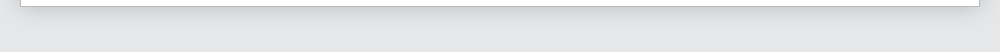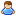Login Products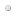SalesSupportDownloadsAbout
 Home » Technical Support » ElevateDB Technical Support » Product Manuals » ElevateDB Version 2 SQL Manual » Types » Interval Types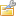Interval Types

Interval types are used to represent the difference between two dates, times, or timestamps. There are two classes of interval values:

Year-Month Intervals
Day-Time Intervals

These two classes are not type-compatible and cannot be assigned to each other or cast between each other.

 Type Description
 INTERVAL YEAR An interval value representing the number of years between two date values. INTERVAL MONTH An interval value representing the number of months between two date values. INTERVAL YEAR TO MONTH An interval value representing the number of years and months (remainder) between two date values. INTERVAL DAY An interval value representing the number of days between two date values. INTERVAL HOUR An interval value representing the number of hours between two time values. INTERVAL MINUTE An interval value representing the number of minutes between two time values. INTERVAL SECOND An interval value representing the number of seconds between two time values. INTERVAL MSECOND An interval value representing the number of milliseconds between two time values. INTERVAL DAY TO HOUR An interval value representing the number of days and hours between two timestamp values. INTERVAL DAY TO MINUTE An interval value representing the number of days, hours, and minutes between two timestamp values. INTERVAL DAY TO SECOND An interval value representing the number of days, hours, minutes, and seconds between two timestamp values. INTERVAL DAY TO MSECOND An interval value representing the number of days, hours, minutes, seconds, and milliseconds between two timestamp values. INTERVAL HOUR TO MINUTE An interval value representing the number of hours and minutes between two time values. INTERVAL HOUR TO SECOND An interval value representing the number of hours, minutes, and seconds between two time values. INTERVAL HOUR TO MSECOND An interval value representing the number of hours, minutes, seconds, and milliseconds between two time values. INTERVAL MINUTE TO SECOND An interval value representing the number of minutes and seconds between two time values. INTERVAL MINUTE TO MSECOND An interval value representing the number of minutes, seconds, and milliseconds between two time values. INTERVAL SECOND TO MSECOND An interval value representing the number of seconds and milliseconds between two time values.

Literals
Interval literals are specified using the following formats:

 Format Description
 INTERVAL 'Y' YEAR Y is the years. INTERVAL 'M' MONTH M is the months. INTERVAL 'Y-M' YEAR TO MONTH Y is the years and M is the months. INTERVAL 'D' DAY D is the days. INTERVAL 'H' HOUR H is the hours. INTERVAL 'M' MINUTE M is the minutes. INTERVAL 'S' SECOND S is the seconds. INTERVAL 'Z' MSECOND Z is the milliseconds. INTERVAL 'D H' DAY TO HOUR D is the days and H is the hours. INTERVAL 'D H:M' DAY TO MINUTE D is the days, H is the hours, and M is the minutes. INTERVAL 'D H:M:S' DAY TO SECOND D is the days, H is the hours, M is the minutes, and S is the seconds. INTERVAL 'D H:M:S.Z' DAY TO MSECOND D is the days, H is the hours, M is the minutes, S is the seconds, and Z is the milliseconds. INTERVAL 'H:M' HOUR TO MINUTE H is the hours and M is the minutes. INTERVAL 'H:M:S' HOUR TO SECOND H is the hours, M is the minutes, and S is the seconds. INTERVAL 'H:M:S.Z' HOUR TO MSECOND H is the hours, M is the minutes, S is the seconds, and Z is the milliseconds. INTERVAL 'M:S' MINUTE TO SECOND M is the minutes and S is the seconds. INTERVAL 'M:S.Z' MINUTE TO MSECOND M is the minutes, S is the seconds, and Z is the milliseconds. INTERVAL 'S.Z' SECOND TO MSECOND S is the seconds, and Z is the milliseconds.

Literal Examples
-- This example specifies a YEAR interval literal

SELECT * FROM Orders
WHERE (OrderDate + INTERVAL '1' YEAR) BETWEEN
DATE '2006-01-01' AND DATE '2006-01-31'

-- This example specifies a DAY interval literal

SELECT * FROM Orders
WHERE (ShipDate - OrderDate) > INTERVAL '2' DAY

-- This example specifies an HOUR interval literal

SELECT * FROM TimeClockEntries
WHERE (PunchOutTime - PunchInTime) > INTERVAL '8' HOUR

SQL 2003 Standard Deviations
The following areas are where ElevateDB deviates from the SQL 2003 standard:

 Deviation Details
 INTERVAL MSECOND Type This is an ElevateDB extension to the day-time interval data types. Interval Precisions ElevateDB does not support specifying the precision of interval values and always uses 4 digits for years, 1-2 digits for months, 1-2 digits for days, 1-2 digits for hours, 1-2 digits for minutes, 1-2 digits for seconds, and 1-3 digits for milliseconds.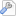More Support Options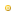Support ForumsFrequently Asked QuestionsTechnical BulletinsTechnical ArticlesIncident ReportsRoadmap